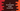# C program to find the length of a linked list recursively# Introduction :

In this C programming tutorial, we will learn how to create a linked list using user input values and how to find out the length of that linked list recursively.

The program will ask the user to enter the values of the list. It will create a linked list using the user given values and calculate the length using a separate recursive function.

## C program :

The C program looks like as below :

``````#include <stdio.h>
#include <stdlib.h>

//3
typedef struct node
{
int value;
struct node *next;
} node;

//11
int getLength(node *head, int size)
{
//12
if (head == NULL)
{
return size;
}
else
{
//13
return getLength(head->next, size + 1);
}
}

int main()
{
//1
int length, i;

//2
printf("Enter size of the list : ");
scanf("%d", &length);

//4
struct node *headNode, *currentNode, *temp;

//5
for (i = 0; i < length; i++)
{
//6
currentNode = (node *)malloc(sizeof(node));

//7
printf("Enter node %d : ", (i + 1));
scanf("%d", &currentNode->value);

//8
if (i == 0)
{
headNode = temp = currentNode;
}
else
{
temp->next = currentNode;
temp = currentNode;
}
}
//9
temp->next = NULL;

//10
printf("Length found using recursion : %d \n", getLength(headNode, 0));
return 0;
}``````

### 1. Create two integer variables length and i. length to store the length of the linked list and i to use in a for loop.

1. Ask the user to enter the length of the list. Read and store it in the length variable.
2. Create one structure node. It can contain one integer value and one address of a similar structure.
3. Create three variables of type node : headNode,currentNode and temp.
4. Run one for loop to read the user inputs. This loop will run for length number of times.
5. Using malloc, assign memory to the node currentNode.
6. Ask the user to enter the value for the current node. Read it and store it in the value field of currentNode.
7. Check if this is the first node or not. If first node, assign the value of currentNode to headNode. Also, assign it to temp. Else, assign it to temp->next and make currentNode as temp.
8. After the loop is completed, assign the next of temp as NULL.
9. Finally, call the method getLength to get the length of the list recursively.
10. This method takes two parameters: the start node and the current size. It will keep increasing the value of current size and return it when the recursion will complete.
11. Check if the current node is NULL or not. If yes, return the current size passed to the method.
12. Else, call the same method recursively with the next of the node and incrementing the size by 1.

### Sample Output :

``````Enter size of the list : 5
Enter node 1 : 1
Enter node 2 : 2
Enter node 3 : 4
Enter node 4 : 6
Enter node 5 : 7
Length found using recursion : 5

Enter size of the list : 4
Enter node 1 : 1
Enter node 2 : 5
Enter node 3 : 4
Enter node 4 : 6
Length found using recursion : 4

Enter size of the list : 3
Enter node 1 : 1
Enter node 2 : 2
Enter node 3 : 3
Length found using recursion : 3``````